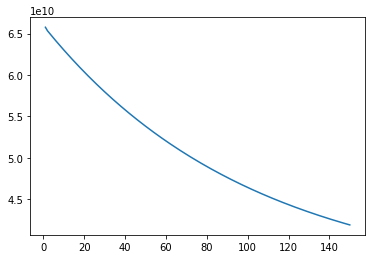# Step 1: Reading the Data

In this example, I will be reading the data from a csv file which is usually the preferred file format for storing and reading data.

`import pandas as pdimport numpy as npfrom sklearn.preprocessing import MinMaxScalerimport matplotlib.pyplot as pltdata_frame = pd.read_csv('kc_house_data.csv')X = data_frame[['bedrooms', 'sqft_living']]y = data_frame['price']X = X.to_numpy()y = y.to_numpy()`

# Step 2: Scaling the Data

Before performing Linear Regression, you should always scale all your x values between 0 and 1 because it eases the calculations and it produces more accurate results.

`scaler = MinMaxScaler()scaler.fit(X)X = scaler.transform(X)`

# Step 3: Declaring Constants

In the last article, I discussed how the step size for Gradient Descent is dependent on the learning rate We will declare the learning rate variable here and also the number of training examples.

`learning_rate = 1 # This decides our step size in Gradient Descentm = len(X) # This is the number of training examples`

# Step 4: Making the Cost Function

In my previous article, I also talked about the cost or the loss function which is used to determine the error in the model’s predictions. The preferred cost function for Linear Regression is Mean Squared Error.

`def cost_function(theta, X, y):    sum = 0    for index, x_val in enumerate(X):        prediction = theta+theta*x_val+theta*x_val        difference = prediction-y[index]        difference_square = difference**2        sum+=difference_square    error = (sum)/(2*m)    return error`

# Step 5: Making the Derivative Functions

To optimize our cost function, we can use Gradient Descent which is a good algorithm for optimization.

`def d_theta_0(t):    answer = 0    for index, x_value in enumerate(X):        pred = t+t*x_value+t*x_value        diff = pred-y[index]        answer+=diff    answer = (answer)/(m)    return answerdef d_theta_1(t):    answer = 0    for index, x_value in enumerate(X):        pred = t+t*x_value+t*x_value        diff = pred-y[index]        diff_2 = diff*x_value        answer+=diff_2    answer = (answer)/(m)    return answerdef d_theta_2(t):    answer = 0    for index, x_value in enumerate(X):        pred = t+t*x_value+t*x_value        diff = pred-y[index]        diff_2 = diff*x_value        answer+=diff_2    answer = (answer)/(m)    return answer# The parameter t for all of these function means the vector theta which contains the parameters for our hypothesis function`

# Step 6: Training the Model

Now its finally time to train our model and perform the linear regression. We will first initialize our parameters vector for our hypothesis function randomly and then we will improve the parameters using gradient descent. We can run as many gradient descent iterations as we want but after a while, the decrease in loss will be really less and we might overfit to the data. The number of iterations is called epochs in machine learning.

`epochs = 150loss_history = [] # This is for tracking the loss at each epoch so we can plot the loss later onparameters = np.random.rand(3,1) # This will be create a vector of 3 random parametersfor i in range(epochs):    p = parameters.copy() # We make a copy of the parameters so that we can assign each parameter simultaneously    parameters-=(learning_rate*d_theta_0(p))    parameters-=(learning_rate*d_theta_1(p))    parameters-=(learning_rate*d_theta_2(p))    loss = cost_function(parameters, X, y)    loss_history.append(loss)`
`plt.plot(range(1, 151), loss_history)plt.show()`My graph of the loss history over 150 epochs
`with open('parameters.txt', 'w') as f:     f.write(str(parameters)+'\n'+str(parameters)+'\n'+str(parameters))`

# Step 7: Predicting using the model

We can now finally predict prices using the model using our parameters!

`def predict_price(sqft, no_of_bedrooms):    price = parameters+parameters*no_of_bedrooms+parameters*sqft    print(price)`

# Conclusion

Linear Regression is a very useful algorithm in predicting continuous values. This python implementation shows you exactly how it works. I would recommend running this code on a machine with a GPU or Google Colab because Google Colab provides a GPU. Machine Learning is very computationally expensive and GPUs are perfect for the number of operations that need to be performed in machine learning. Try this out and try using another dataset to practice linear regression.

--

--